# Physics - Classical Mechanics - Exercises around Damped and Forced Oscillation

in STEMGeeks4 months ago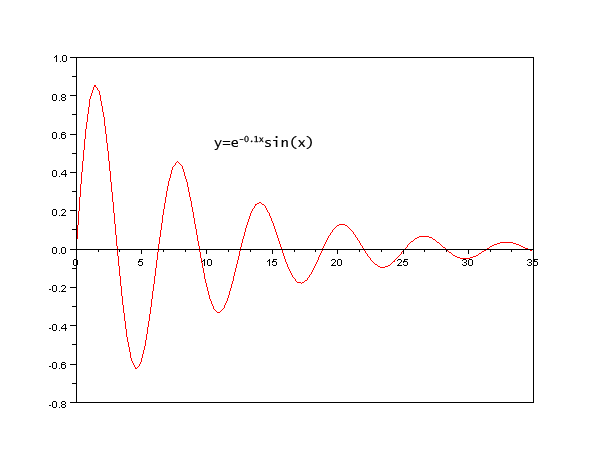[Image1]

## Introduction

Hey it's a me again @drifter1!

Today we continue with Physics, and more specifically the branch of "Classical Mechanics" in order to get into Exercises around Damped and Forced Oscillation.

So, without further ado, let's get straight into it!

## Example 1: Damped Oscillation

Let's consider a mass of 0.2 Kg is attached to one side of a spring with constant 320 N/m. The system starts oscillating, while a damping force of the form F = -bv acts upon it.

Calculate:

• The period of oscillation (T) for damping constant b = 8 Kg/s
• The value of b for critical damping
• The value of b for an initial amplitude of 0.5 m and decrease to 0.1 m in 3 sec

### Solution

The period is related to the angular frequency (ω), which for the corresponding values can easily be calculated as follows: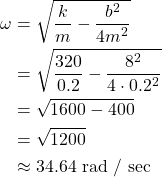And so, for b = 8 Kg/s the period is: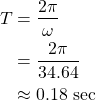Now for the next question, as we know critical damping is achieved for a damping constant of: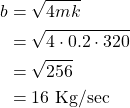The last question is a little more complicated. We will have to use the motion equation: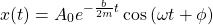Everything is then known except for the damping constant. Of course, the initial phase is φ = 0, because we have maximum positive displacement. Because the angular frequency (ω) is unknown, we will also have to substitute with the square root equation, where again only the value of b is missing.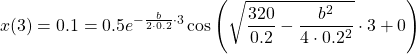Well, what remains now is simply solving this "abomination" for b and we're done. Using wolfram alpha, the numerical result that was returned is: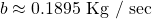## Example 2: Forced Oscillation

Let's continue on from the previous example, by applying an external driving force Fd upon the system. Consider the damping constant b = 8 Kg/s for the calculations.

Calculate:

• The maximum value of the driving force (F0) if the amplitude of the system (after reaching steady state) is A = 1 m and the driving frequency is ωd = 20 rad /sec
• The maximum possible amplitude that the oscillation can reach for that same maximum driving force

### Solution

Let's first calculate the natural frequency of the system: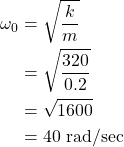The driving frequency is smaller and thus we expect the maximum possible amplitude to be larger for the same maximum driving force.

Substituting, the known values into the amplitude equation, the maximum driving force is: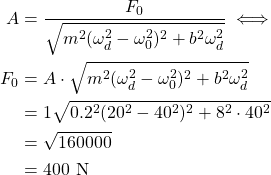The maximum amplitude is achieved for ωd = ω0, which turns the first term in the square root into zero, and yields: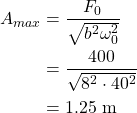As expected the amplitude is larger...

## RESOURCES:

### References

Previous parts...

## Final words | Next up

And this is actually it for today's post!

Next time we will get into Chaotic Oscillation (or Chaos)...

See ya!Keep on drifting!

Posted with STEMGeeks